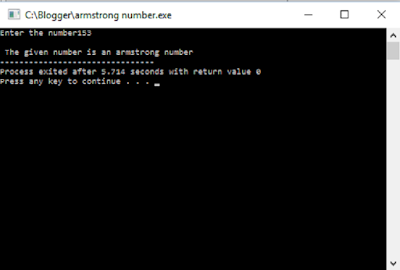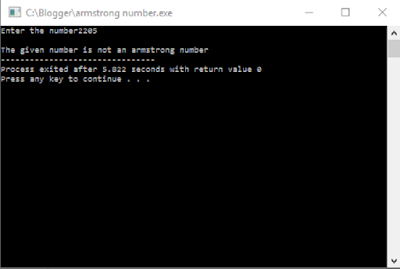## Sunday, 14 February 2016

### Write a C program to check if the given number is a armstrong number or not? - Online Rockers

Greetings viewers, We  are back.Today our topic is going to be Armstrong number.

### What is Armstrong number?

Any guesses ?  Consider a number 371 . When you split each digit , cube them and add them and you get the same digit , then it is called Armstrong number.

3^3 = 27

7^3=343

1^3=1

(3)^3 + (7)^3 + (1)^3 = 27 + 343 + 1 = 371

And thus you get the same number again ( No. 371 )

0

1

153

370

371

407

### How to write a C program for Armstrong number?

Well , this is so simple . The main idea is that you need to

• You need to split each number using modulus operator.
• Cube the number and add it .
•  Check if the number is same as that of initial number.
• If same , it is called Armstrong number.

Note : In order to compile a C program , you need a C compiler. You can download Dev C/C++ compiler here for free.

### Source code:

#include<stdio.h>
int main()
{
int a,num,b,sum=0;
printf("Enter the number");
scanf("%d",&num);                       //accepting number from user
a=num;
while(num>0)
{                                                      //loop to find cube and sum of digits
b=num%10;
sum=sum+(b*b*b);
num=num/10;
}
if(a==sum)                                   //loop to check if it is an armstrong number
{
printf("\n The given number is an armstrong number");
}
else
{
printf("\nThe given number is not an armstrong number");
}
return 0;
}

### Output:

#### Sample output 1:#### Sample output 2:### Check out other C programs:

We welcome your suggestions and feedback.